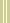July 23, 2001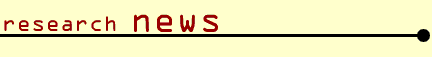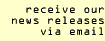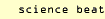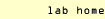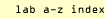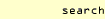BERKELEY, CA � David H. Bailey, chief technologist of the Department of Energy's National Energy Research Scientific Computing Center (NERSC) at Lawrence Berkeley National Laboratory, and his colleague Richard Crandall, director of the Center for Advanced Computation at Reed College, Portland, Oregon, have taken a major step toward answering the age-old question of whether the digits of pi and other math constants are "random." Their results are reported in the Summer 2001 issue of Experimental Mathematics.

Pi, the ubiquitous number whose first few digits are 3.14159, is irrational, which means that its digits run on forever (by now they have been calculated to billions of places) and never repeat in a cyclical fashion. Numbers like pi are also thought to be "normal," which means that their digits are random in a certain statistical sense.

Describing the normality property, Bailey explains that "in the familiar base 10 decimal number system, any single digit of a normal number occurs one tenth of the time, any two-digit combination occurs one one-hundredth of the time, and so on. It's like throwing a fair, ten-sided die forever and counting how often each side or combination of sides appears."

Pi certainly seems to behave this way. In the first six billion decimal places of pi, each of the digits from 0 through 9 shows up about six hundred million times. Yet such results, conceivably accidental, do not prove normality even in base 10, much less normality in other number bases.

In fact, not a single naturally occurring math constant has been proved normal in even one number base, to the chagrin of mathematicians. While many constants are believed to be normal -- including pi, the square root of 2, and the natural logarithm of 2, often written "log(2)" -- there are no proofs.

The determined attacks of Bailey and Crandall are beginning to illuminate this classic problem. Their results indicate that the normality of certain math constants is a consequence of a plausible conjecture in the field of chaotic dynamics, which states that sequences of a particular kind, as Bailey puts it, "uniformly dance in the limit between 0 and 1" -- a conjecture that he and Crandall refer to as "Hypothesis A."

"If even one particular instance of Hypothesis A could be established," Bailey remarks, "the consequences would be remarkable" -- for the normality (in base 2) of pi and log(2) and many other mathematical constants would follow.

This result derives directly from the discovery of an ingenious formula for pi that Bailey, together with Canadian mathematicians Peter Borwein and Simon Plouffe, found with a computer program in 1996. Named the BBP formula for its authors, it has the remarkable property that it permits one to calculate an arbitrary digit in the binary expansion of pi without needing to calculate any of the preceding digits. Prior to 1996, mathematicians did not believe this could be done.

The digit-calculation algorithm of the BBP formula yields just the kind of chaotic sequences described in Hypothesis A. Says Bailey, "These constant formulas give rise to sequences that we conjecture are uniformly distributed between 0 and 1 -- and if so, the constants are normal."

Bailey emphasizes that the new result he and Crandall have obtained does not constitute a proof that pi or log(2) is normal (since this is predicated on the unproven Hypothesis A). "What we have done is translate a heretofore unapproachable problem, namely the normality of pi and other constants, to a more tractable question in the field of chaotic processes."

He adds that "at the very least, we have shown why the digits of pi and log(2) appear to be random: because they are closely approximated by a type of generator associated with the field of chaotic dynamics."

For the two mathematicians, the path to their result has been a long one. Bailey memorized pi to more than 300 digits "as a diversion between classroom lectures" while still a graduate student at Stanford. In 1985 he tested NASA's new Cray-2 supercomputer by computing the first 29 million digits of pi. The program found bugs in the Cray-2 hardware, "much to the consternation of Seymour Cray."

Crandall, who researches scientific applications of computation, suggested the possible link between the digits of pi and the theory of chaotic dynamic sequences.

While other prominent mathematicians in the field fear that the crucial Hypothesis A may be too hard to prove, Bailey and Crandall remain sanguine. Crandall quotes the eminent mathematician Carl Ludwig Siegel: "One cannot guess the real difficulties of a problem before having solved it."

Among the numerous connections of Bailey's and Crandall's work with other areas of research is in the field of pseudorandom number generators, which has applications in cryptography.

"The connection to pseudorandom number generators is likely the best route to making further progress," Bailey adds. "Richard and I are pursuing this angle even as we speak."

For more about the normality of pi and other constants, visit David Bailey's website. The BBP algorithm for calculating binary digits of pi was found using the PSLQ algorithm developed by Bailey and mathematician-sculptor Helaman Ferguson; it is discussed at Bailey's website and also in the Fall 2000 issue of Berkeley Lab Highlights.

The Berkeley Lab is a U.S. Department of Energy national laboratory located in Berkeley, California. It conducts unclassified scientific research and is managed by the University of California.

Contact information: